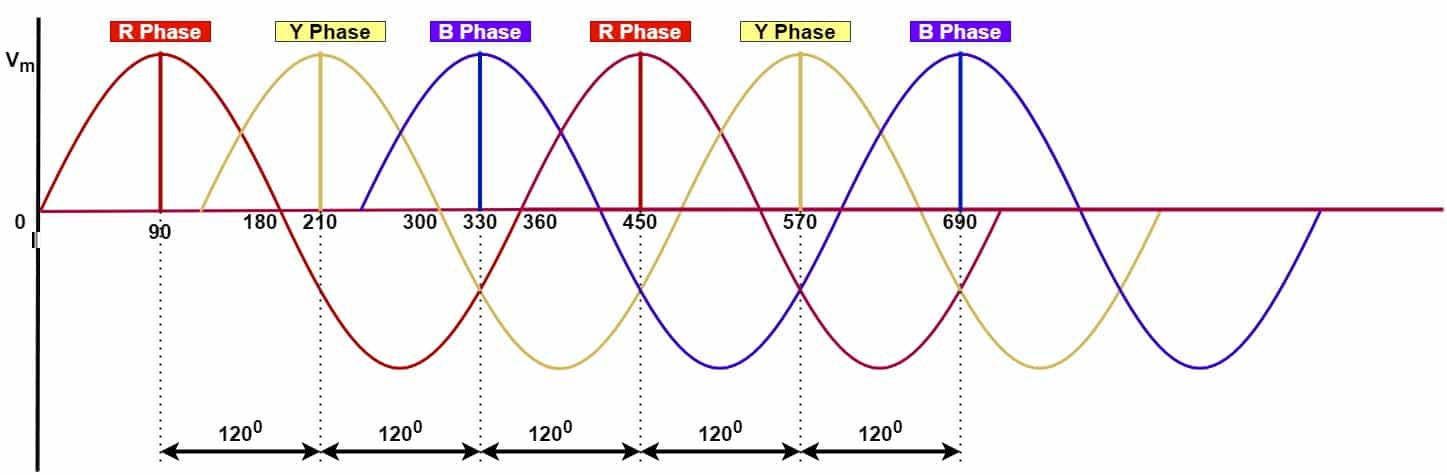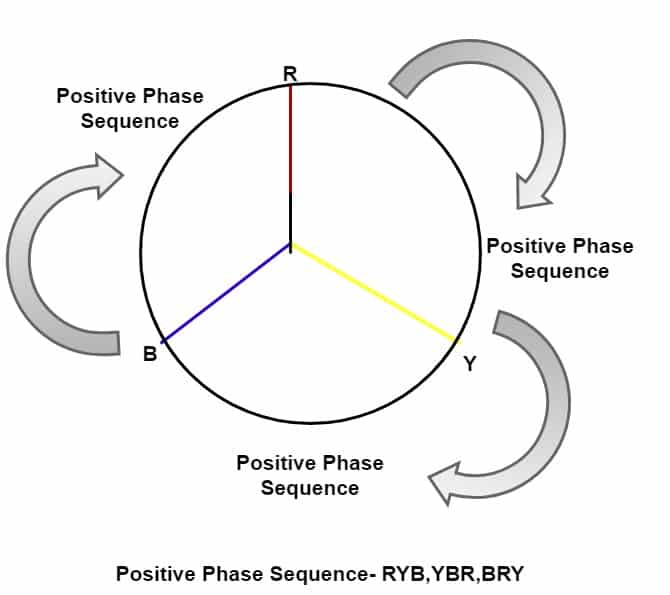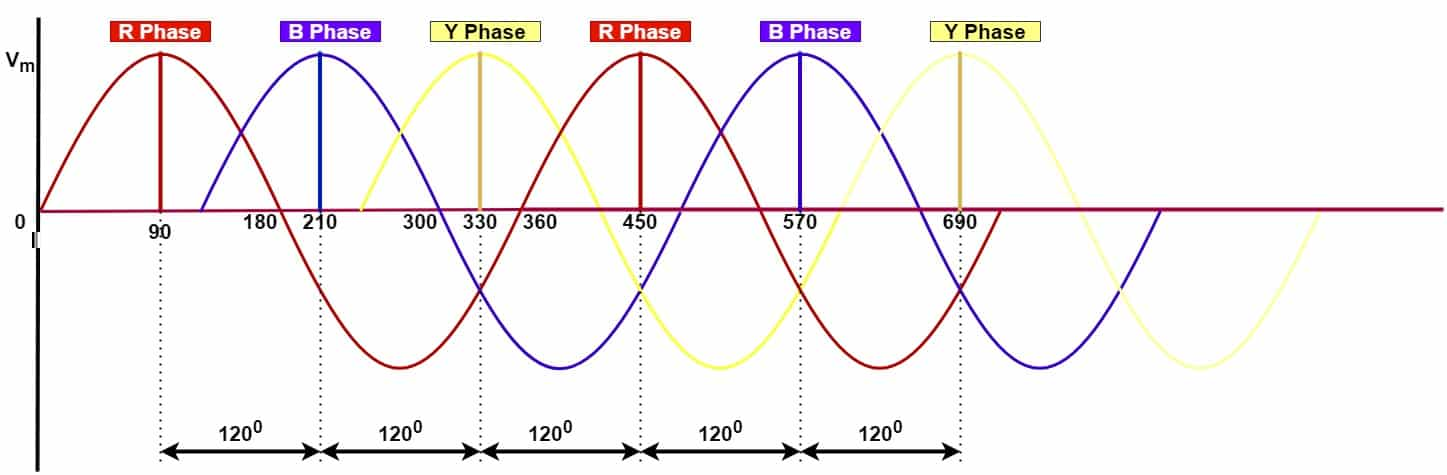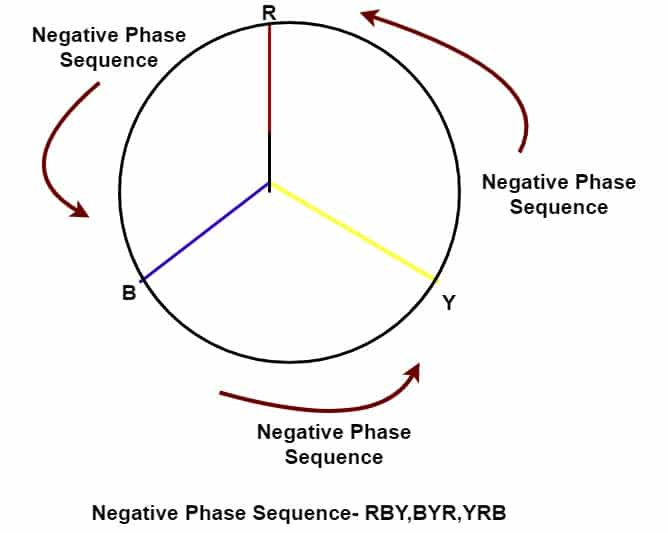# What is a Phase Sequence & Its Significance

In a three-phase power system, the order in which each phase voltages attain a positive peak value is called phase sequence. The phase sequence is also known as phase rotation.  if the order of phase sequence in 3 phase system is denoted as RYB, then the R phase attains its positive peak value first, after 120 degrees the Y phase attains its positive peak value, and after 120 degrees B phase attains its positive peak value.In the above-given waveform, the phase sequence or phase rotation is RYB. R,Y, and B phase attains positive peak value after 90, 210, 310 degrees respectively.

### Positive SequenceThe other order sequence is YBR and BRY. In the above-shown waveform, the order of attaining maximum voltage is RYB, YBR, and BRY. This order of attaining maximum voltage shows a Positive phase sequence which rotates in a clockwise direction.### Negative SequenceThe order of attaining peak positive voltage is RBY. The other order of attaining peak positive voltage is BYR and YRB. In the above-shown waveform, the order of attaining maximum voltage is  RBY, BYR, and YRB and it shows Negative phase sequence or Negative phase rotation which rotates in the anti-clockwise direction. The below figure shows the negative phase rotation.### Importance of Phase Sequence

The phase sequence is essential because of the following reasons.

1. The positive phase sequence is RYB, YBR, BRY rotates in the clockwise direction. If the stator of the motor receives a three-phase supply in the above-said phase sequence, then the motor rotates in the same direction for the three sets of order RYB, YBR and BRY. The RBY, BYR, YRB rotates clockwise and the motor will rotate in the opposite direction if feed the supply of anti-clockwise sequence voltage.

2. The parallel operation of the transformers if both transformers have the same vector group. It means the phase rotation of both the transformer is the same. Both the transformer’s phase rotations should be RYB or YBR or BRY.

3. The parallel operation of the generators is possible when the generated voltages of both the generator have the same phase rotation. Both the generators’ phase rotations should be RYB or YBR or BRY.
4. When two power sources need to operate in parallel, the phase rotation of both the sources should be the same. The synchronizing check relay permits if the phase rotation, frequency and voltage of the two power sources are the same. Here phase rotation of both the sources should be RYB or YBR or BRY. One source phase rotation is RYB and another source phase rotation is YBR means both the phase rotation is clockwise. But,  we should not synchronize the power sources in this case.

The phase sequence indicator is very useful for knowing the phase rotation, whether it has positive phase rotation or negative phase rotation.

Related Posts

1. What is Peak Value, Average Value and RMS Value?
2. Parallel Operation of Transformers
3. What is Crest Factor or Peak Factor?
4. What is Form Factor? Form Factor Formula & its Derivation